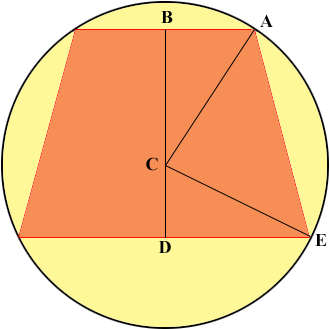SEARCH HOMEMath Central Quandaries & QueriesQuestion from Abby, a student: An isosceles trapezoid whose bases have lengths 12 and 16 is inscribed in a circle of radius 10. The center of the circle lies in the interior of the trapezoid. Find the area of the trapezoidHi Abby,

In my diagram C is the center of the circle and B is the midpoint of the side of the trapezoid of length 12.Triangle ABC is a right triangle (why?), the length of CA is 10 and the length of AB is 6. Use Pythagoras theorem to find the length of BC. Use a similar argument to find the length of CD.

Now you know the lengths of the parallel sides of a trapezoid and the distance between the parallel sides. What is its area?

PennyMath Central is supported by the University of Regina and The Pacific Institute for the Mathematical Sciences.